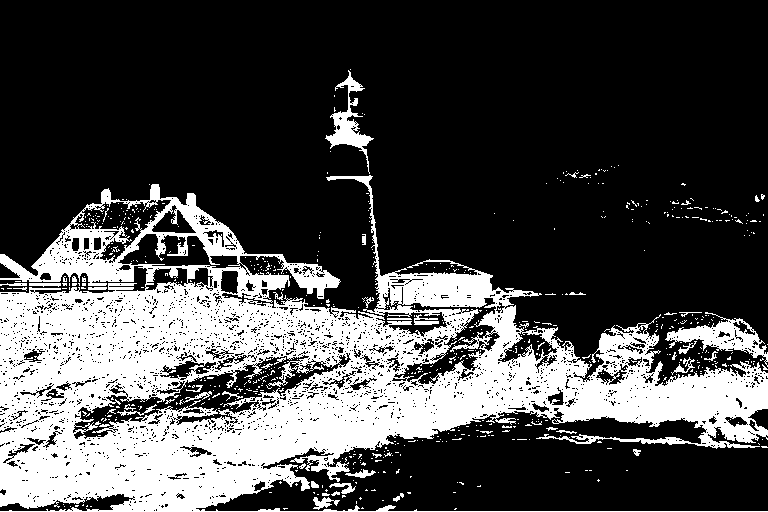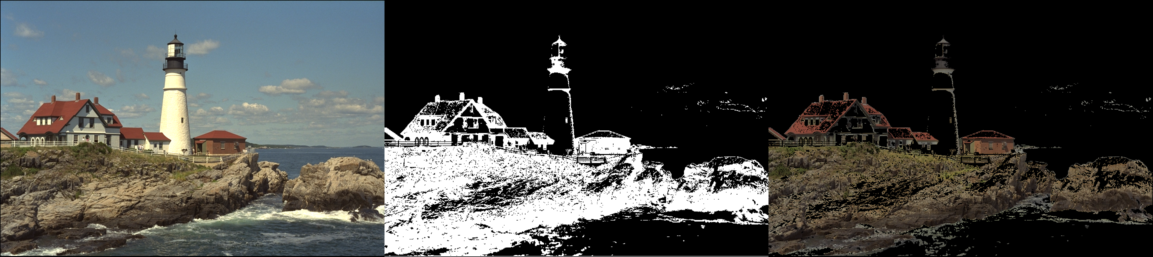# RGB to HSV and thresholdingThis example illustrates how RGB to HSV (Hue, Saturation, Value) conversion can be used to facilitate segmentation processes.

using Images, TestImages, LinearAlgebra

rgb_img = testimage("lighthouse")
hsv_img = HSV.(rgb_img)
channels = channelview(float.(hsv_img))
hue_img = channels[1,:,:]
value_img = channels[3,:,:]
saturation_img = channels[2,:,:]

A simple segmentation of the image can then be effectively performed by a mere thresholding of the HSV channels.

mask = zeros(size(hue_img))
h, s, v = 80, 150, 150
for ind in eachindex(hue_img)
if hue_img[ind] <= h && saturation_img[ind] <= s/255 && value_img[ind] <= v/255
binary_img = colorview(Gray, mask)segmented_img = mask .* rgb_img
hcat(rgb_img, binary_img, segmented_img)